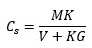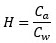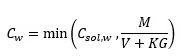## December 14, 2020

### GoldSim Contaminant Transport Module Online Course, Excerpt #5: Chemical Processes Controlling Mass Transport

Posted by Rick Kossik

This is the fifth installment of short excerpts from the online CT Course that is currently under development. These excerpts are all, by necessity, from the conceptual parts of the Course, as those parts that are "hands-on" don't necessarily work well in a blog format.  However, there is plenty of conceptual matter to discuss (the Course is very detailed and extensive), so I'm hoping you will still find these excerpts useful.

The first excerpt simply provided the outline describing how the Course will be organized.  The second excerpt  discussed the key decisions required before starting to build a contaminant transport model. The third excerpt discussed the problem of uncertainty in contaminant transport models and the underlying philosophy of the GoldSim Contaminant Transport Module. The fourth excerpt provided an overview of the physical processes controlling mass transport (advection, dispersion and diffusion).

This excerpt provides an overview of the chemical processes controlling fate and transport.

In addition to the physical processes described in the previous excerpt, solutes can be impacted by chemical processes. This can include both abiotic and biologically-mediated reactions in which the chemical is transformed or converted to other chemicals, such as radioactive decay or degradation of an organic chemical by bacteria, reactions that cause a solute to precipitate out of solution and form a solid, the sorption of a chemical from water onto a solid, and the volatilization of a chemical from water to air.

When modeling a chemical process, it is important to differentiate between whether we are representing the process from a standpoint of kinetics or equilibrium. Kinetics describe the rate at which a process takes place.  Equilibrium describes the system in terms of the final expected outcome. Equilibrium models of a chemical process are appropriate if the process is rapid compared to other processes of interest.

For example, a radioisotope that was slowly decaying to a daughter (and was not ingrowing from a parent) could not be treated using an equilibrium approach, since equilibrium (in which the species has decayed completely to some other species) may not be reached for thousands of years, at which point the “expected outcome” would be that it is effectively gone. The kinetics of the reaction would need to be specifically modelled. On the other hand, the partitioning of a metal between water and a porous medium within a flowing aquifer may perhaps be considered rapid (relative to the flow of water) and hence could be treated using an equilibrium approach.

Within a GoldSim model, you will typically treat some processes by explicitly modeling the kinetics (e.g., radioactive decay) while treating other processes using an equilibrium approach (e.g., sorption onto porous media).

Reactions

Let’s first consider the process of abiotic or biologically-mediated chemical reactions that degrade a chemical (and produce reaction products that you may or may not be interested in subsequently tracking). Chemical reactions can be described in terms of their order. A first-order reaction is one in which the rate is proportional to the chemical’s concentration raised to the first power. Radioactive decay is a classic example of a first-order reaction. To see how such a reaction would be treated mathematically, let’s consider the simple example with two tanks that we discussed in the previous excerpt:Recall that the tanks are always completely and instantaneously mixed and water flows through the tanks at a constant volumetric rate of Q (such that the volumes remain constant).  Initially, both tanks have no chemical mass in them, but at some point we start to inject mass into the inflow to Tank 1 (such that the inflow has a constant concentration of S). The system includes only the two tanks (and the pipe connecting them). There is a boundary upstream of Tank 1 (the mass flowing in is an external forcing function) and there is a boundary downstream of Tank 2 (we no longer track the mass after it leaves Tank 2).

However, let’s now consider that the chemical being introduced (we’ll call it A) decays with a rate constant of λ (dimensions of inverse time).  Moreover, let’s assume that when it decays it produces a daughter product (named B).

In the previous excerpt, the two tank system was represented by two equations.  However, since we have two species being modeled, we now have four equations (two for each species):In these equations the various subscripts now refer to both the tank (1 or 2) and the species (A or B). The terms in red represent the reaction. The first two equations describe the rate of change of A in the two tanks, and are identical to the equations presented in the previous excerpt, with the addition of the reaction terms.  In both equations, the rate of change of mass due to this first order reaction is simply -λM.

The last two equations describe the rate of change of B in the two tanks.  They are similar to those for A with several key differences: 1) since only A is being introduced into Tank 1, there is no QS term, and 2) the decay in A results in the ingrowth of B (such that in the reaction term, the rate of addition of B is proportional to the mass of A).

If the reactions involved were higher-order, such that the rate was a function of a concentration to a power and/or the concentration of multiple species (i.e., multiple reactants), the equations would become more complex (e.g., the mass terms might be raised to a power).

GoldSim can very easily handle first-order decay and ingrowth. It can also handle higher-order reactions, but these equations become more complicated for you to represent in a model and more difficult for GoldSim to solve.

Sorption

Another common chemical process impacting the transport of solutes is sorption. Sorption describes a process in which chemicals become associated with solids that are present in the system.  That is, the chemicals partition between water and the solid. This can have a drastic impact on transport.  If the solid is immobile (e.g., sand in an aquifer), sorption effectively decreases the effective concentration and hence the rate of advection is decreased.  Note, however, that a chemical could be sorbed onto a solid that is suspended in a fluid.  Fluid advection then transports the suspended solids (and hence the sorbed mass).

Sorption is a very complex process. For example, it can be reversible (the chemical can move back and forth from fluid to solid depending on the fluid concentration) or non-reversible (once it is sorbed onto the solid, it remains there).  The degree of sorption can also be impacted by a limited number of sorption sites on the solid that are available (such that the solid effectively has a sorption capacity).  The degree of sorption of one chemical may be a function of the other species present in solution (in a very complex way). And the sorption process itself may be slow (i.e., the kinetics of the sorption process may be important).  All of these aspects (and more!) make sorption a complex process to model.  However, one of the simplest (and relatively common) representations of sorption is to assume equilibrium (instantaneous) reversible linear partitioning.  That is, it is assumed that when the chemical enters the compartment of interest (e.g., a well-mixed tank), it is immediately partitioned between the water and the solid according to an equilibrium partition coefficient that describes the ratio of the concentration on the solid to the concentration in the water at equilibrium:In this equation, Cs is the equilibrium concentration associated with the solid (dimensions of mass chemical per mass of solid), Cw is the equilibrium concentration in the water (dimensions of mass chemical per volume of water), and K is the partition coefficient (dimensions of volume water per mass solid).

In the absence of sorption, the concentration of a species can be expressed simply as M/V (where M is the mass and V is the volume of water). Assuming equilibrium partitioning with a single solid, we can express the concentration of a species in the water as:where G represents the mass of solid present.

The corresponding concentration of the species on the solid can be expressed as follows:Note that these equations can easily be expanded to represent equilibrium between multiple fluids and multiple solids.

Considering our two tank problem again (and for simplicity, considering only a single species and no decay), let’s assume that we have some solid in the tank that is suspended and well-mixed throughout.  Let’s assume that the chemical partitions between the water and the solid instantaneously as it enters the tank.  Finally, let’s assume pipes carrying water out of Tank1 and Tank2 have filters, so that none of the solid can leave the tanks (only the dissolved chemical is advected out of the tanks).  In this case, we can write the governing equations as:Of course, if the flow was not filtered, we would need to account for the transport of the chemical that was sorbed onto the suspended solids.  In fact, in this simple case, if all of the solid was suspended (and none was filtered), sorption would have no impact on the mass transport.  It would still have an impact, however, if only a portion of the solid was filtered (or some was not suspended).

GoldSim can very easily handle linear equilibrium sorption onto immobile solids, as well as sorption onto suspended solids that move with the flow.  It can also handle more complex situations (e.g., non-linear sorption in which the degree of partitioning changes with concentration), but like the case for higher-order reactions, these equations become more complicated for you to represent in a model and more difficult for GoldSim to solve.

Volatilization

In the discussion above, we discussed the partitioning of a chemical between a fluid (water) and a solid. However, we can use the same approach to represent the partitioning of a chemical between two fluids. For example, if you had two fluids in a jar, and added a organic chemical, it would partition between the two fluids.  You could do such an experiment with oil and water.  One of the fluids could also be air.

In particular, if the chemical was volatile (had a significant vapor pressure in air) it would tend to volatilize from water into air.  For a chemical present in a lake or some other body of water, this could be a significant loss mechanism.

Mathematically, the partitioning of a volatile chemical between air and water can be described in a similar manner to the partitioning between a solid and water.  That is, it can be described using an equilibrium partition coefficient that describes the ratio of the concentration in the air (in terms of mass chemical/volume air) to the concentration in the water (in terms of mass chemical/volume water):In this case (particularly for volatile organic compounds), the partition coefficient, H, is described as the Henry’s law constant. It has dimensions of volume water per volume of air.

In most situations, it would not be realistic to assume, for example, that an entire water body (e.g., a lake) was at equilibrium with some portion of the atmosphere above the lake.  However, we can still use this partitioning relationship to model the process of volatilization by assuming that the kinetics of the process are controlled by diffusion of the chemical through some small boundary layers (in both the water and the air), and that at the interface itself, the transition from water to air can be described using the Henry’s law constant.

Solubility

In many cases, we are interested in modeling solutes that are present at relatively low concentrations.  In some cases, however, at some locations in the system the solutes may be present at high enough concentrations such that they precipitate out of solution (i.e., exist in both a dissolved state as well as a solid or liquid state). For example, an anion like Ca+2 could precipitate out to form the solid calcite, or an organic compound may be present in such quantities that it cannot totally dissolve and some would be present as a separate liquid phase (that either floats, sinks, or could be suspended as small globules).

In these situations, the solute is still present in water, but its concentration is fixed at its solubility limit. As long as the solid (or in the case of the organic, pure liquid) phase exists, the solute will be present at the solubility limit.  It can drop below the solubility limit (e.g., if all of the solid or liquid phase is removed and the water is subsequently mixed with water at a lower concentration), but (unless under very special circumstances) cannot exceed the solubility limit.

This process can obviously have an enormous impact on mass transport, as it has the impact of limiting the dissolved concentration (and hence the advective flux) of contaminants through a system. In fact, solubility plays the key role in many treatment processes (chemicals are added to cause precipitation and then the solid is removed from the system). In many systems, the concentration of the contaminant at its source (e.g., in an engineered disposal facility) is effectively fixed by solubility constraints.  Ignoring these in a model could overestimate the actual concentration by many orders of magnitude.

Hence, it is important to account for this process if your solute concentrations would otherwise exceed solubilities.  As you can imagine, however, modeling the chemical processes controlling solubility can be quite complex.  For example, a particular metal may form multiple solids and different dissolved complexes, depending on the other solutes present in solution as well as the chemical characteristics of the water (e.g., pH, redox).

For some applications, it may be necessary to model the various geochemical processes in great detail. GoldSim itself cannot carry out detailed geochemical calculations (e.g., it does not have a database of geochemical data, nor does it “understand” the various chemical reactions). You could, however, dynamically link GoldSim to a tool that specifically does so. (Doing so, however, is quite complex and would require very advanced GoldSim skills).

In many cases, however, such an approach may not be necessary or appropriate at all. A simpler approach, such as specifying a solubility value for each species that you are modelling (representing it as a constant, or describing how it changes temporally and spatially) may be appropriate. GoldSim does support this approach.

With such an approach, in all of the equations we have described above, when accounting for solubility constraints, the concentration in water (assuming in this example, we also have sorption occurring) is modified as follows:In this equation, Csol,w is the specified solubility in water. (It should be noted that GoldSim is actually more sophisticated than simply assigning a solubility to each species being simulated.  If you are modeling multiple radioisotopes, the solubility applies to all the isotopes of a particular element, not to the individual isotopes, and GoldSim accounts for this.)